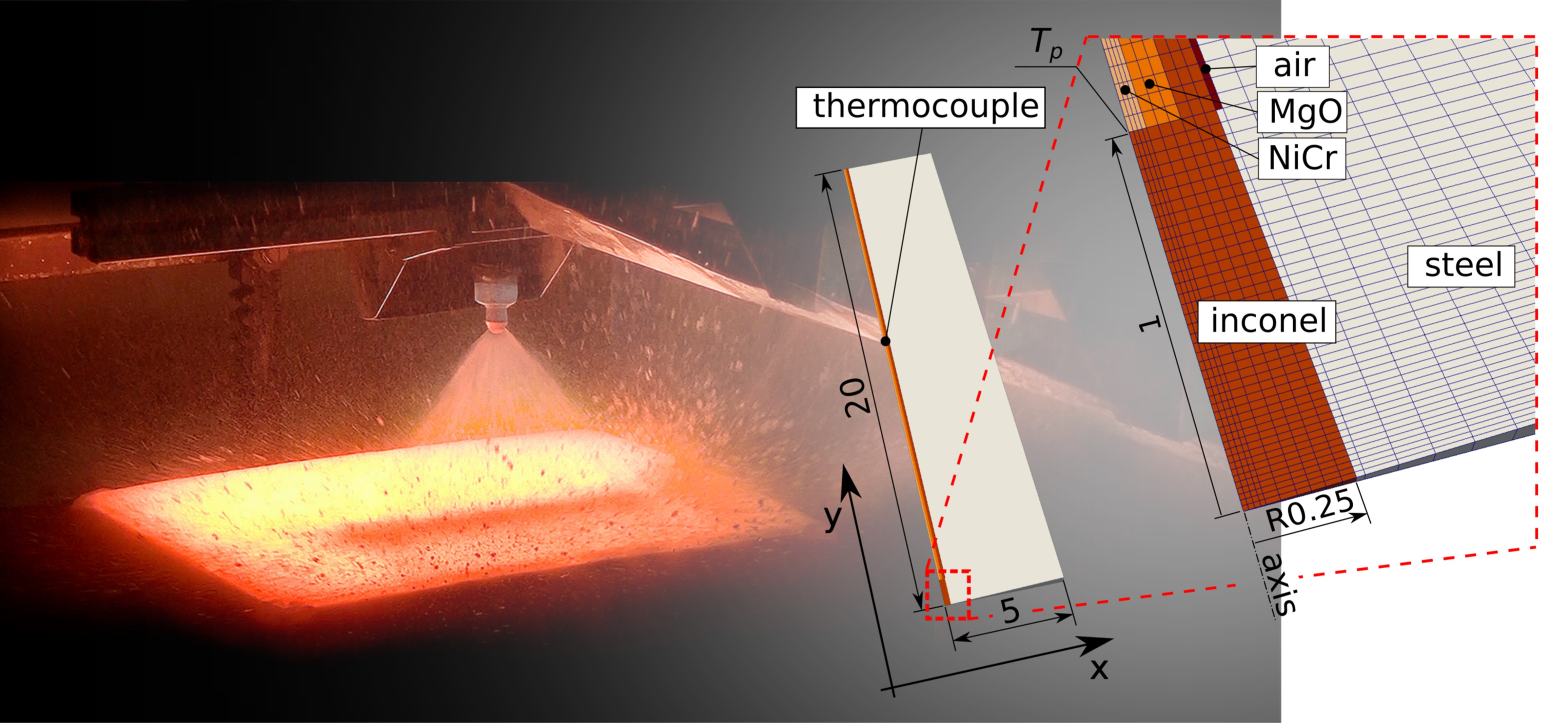# A SEQUENTIAL INVERSE HEAT CONDUCTION PROBLEM IN OPENFOAM®

## Authors

• Jan Bohacek Brno University of Technology
• Jan Kominek Brno University of Technology
• Alexander Vakhrushev Montanuniversitaet Leoben
• Ebrahim Karimi-Sibaki Montanuniversitaet Leoben
• Taewoo Lee Arizona State University

## Keywords:

Inverse Heat Conduction Problem, heat transfer, spray cooling, OpenFOAM, optimization, BOBYQA

## Abstract

The solution of the inverse heat conduction problem (IHCP) is commonly found with the sequential algorithm known as the function specification method with explicit updating formulas and sensitivity coefficients of heat flux. This paper presents a different approach namely a direct mathematical optimization of minimizing the least squares norm between experimental data and simulation. A CFD open-source code OpenFOAM is used together with NLOPT and DLIB optimization libraries. To guarantee credibility of the simulation tool developed herein, real experimental data is used from spray cooling of a fast-moving hot steel plate. As the IHCP is inherently an ill-posed problem, the proposed sequential algorithm is stabilized using future time stepping and thereof the optimal number is explained. An assumption about the profile of thermal boundary condition during future steps must be made. It is shown that assuming a linear change of the heat transfer coefficient during each sequence of future time steps yields more accurate results than setting a constant value. For the problem size considered with less than 10k cells, the preconditioned conjugate gradient (FDIC) linear solver converges faster than the multigrid solver (GAMG). However, the latter performs better as the accuracy is concerned. Concerning the best choice of minimizer, the BOBYQA algorithm (quadratic approximation) is found superior to other methods. The proposed IHCP solver is compared with the well-established one.